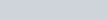In an A.P., if the 5th and 12th terms are 30 and 65 respectively,
Question:

In an A.P., if the 5th and 12th terms are 30 and 65 respectively, what is the sum of first 20 terms?

Solution:

In the given problem, let us take the first term as a and the common difference d

Here, we are given that,

$a_{5}=30$………..(1)

$a_{12}=65$………(2)

Also, we know,

$a_{n}=a+(n-1) d$

For the 5th term (n = 5),

$a_{5}=a+(5-1) d$

$30=a+4 d$ (Using 1)

$a=30-4 d$ …………….(3)

Similarly, for the 12th term (n = 12),

$a_{12}=a+(12-1) d$

$65=a+11 d$ (Using 2)

$a=65-11 d$ ……………(4)

Subtracting (3) from (4), we get,

$a-a=(65-11 d)-(30-4 d)$

$0=65-11 d-30+4 d$

$0=35-7 d$

$7 d=35$

$d=5$

Now, to find a, we substitute the value of d in (4),

$a=30-4(5)$

$a=30-20$

$a=10$

So, for the given A.PSo, to find the sum of first 20 terms of this A.P., we use the following formula for the sum of n terms of an A.P.,

$S_{n}=\frac{n}{2}[2 a+(n-1) d]$

Where; a = first term for the given A.P.

d = common difference of the given A.P.

= number of terms

So, using the formula for n = 20, we get,

$S_{20}=\frac{20}{2}[2(10)+(20-1)(5)]$

$=(10)[20+(19)(5)]$

$=(10)[20+95]$

$=(10)$

$=1150$

Therefore, the sum of first 20 terms for the given A.P. is $S_{20}=1150$.